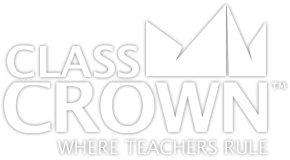## 4th Grade Math Worksheets: Pack 1

Skills included in this pack:
• Interpreting multiplication
• Multiplying or dividing to solve word problems involving multiplication comparison
• Interpreting remainders in division word problems
• Solving word problems with four operations
• Solving two-step problems
• inding factors for whole numbers between 1 and 100
• Identifying factors and multiples
• Identifying prime numbers
• Prime factorization
• Determining if a number is a multiple
• Continuing patterns
• Creating a pattern with a given rule
• Multiplication with multiples of 10
• Examining whole number digit place value
• Using place value to solve multiplication & division problems
• Converting between expanded form and numeric form
• Converting between numeric number and word form
• Comparing numbers using <, >, and =
• Rounding to a given place
• Adding up to four digit numbers
• Subtracting up to four digit numbers
• Multiplying using arrays with factors of 10
• Multiplying using arrays
• Multiplying up to four digits by one digit
• Dividing by one digit with remainders
• Dividing with partial quotients
• Estimating quotients
• Dividing three-digit numbers by one-digit numbers with remainder
• Dividing using visual representation
• Division word problems with and without remainders
+ See More
Free Sample Pages Available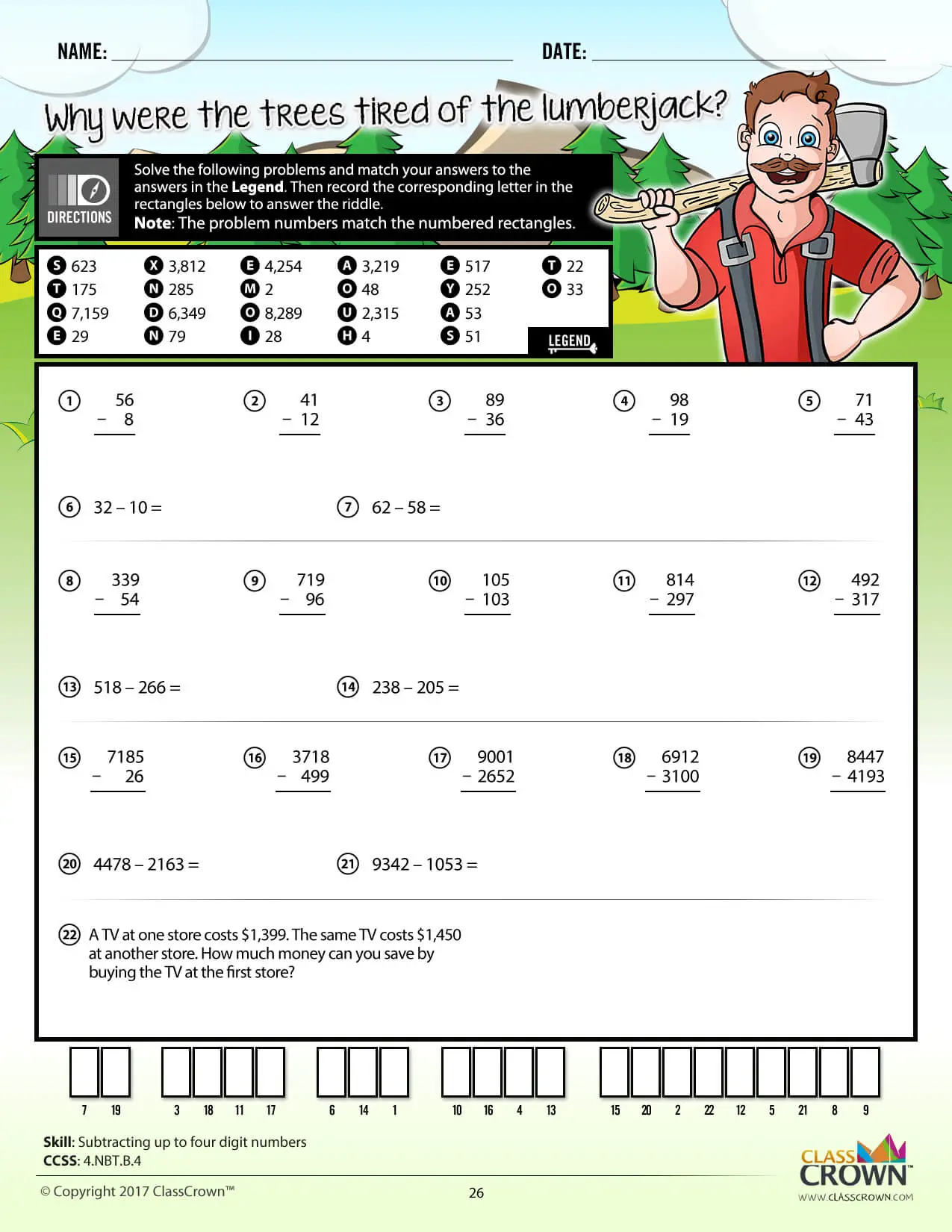## 4th Grade Math Worksheets: Pack 2

Skills included in this pack:
• Finding equivalent fractions
• Finding equivalent fraction patterns
• Simplifying fractions
• Comparing fractions
• Comparing fractions to one half
• Adding parts of a whole - visual
• Adding fractions - numeric & visual
• Adding mixed numbers - visual
• Adding and subtracting improper fractions - same denominator
• Adding mixed numbers - same denominator
• Converting an improper fraction to a mixed number
• Converting a mixed number to an improper fraction
• Subtracting fractions with regrouping - same denominator
• Adding and subtracting fractions, word problems - same denominator
• Multiplying fractions by whole numbers - visual
• Multiplying fractions by whole numbers - visual, bar
• Multiplying unit fractions with a number line
• Creating equivalent unit fraction problems
• Multiplying fractions by whole numbers - word problems
• Expressing a fraction w/ a denominator 10 as a fraction w/ denominator 100
• Finding decimals on a number line
• Converting decimals to fractions
• Finding equivalent fractions and decimals - visual
• Converting fractions to decimals - tenths and hundreds
• Comparing decimals
• Determining greatest and least value
• Ordering decimals
• Ordering money, same dollar amount
• Ordering money, different dollar amounts
+ See More
Free Sample Pages Available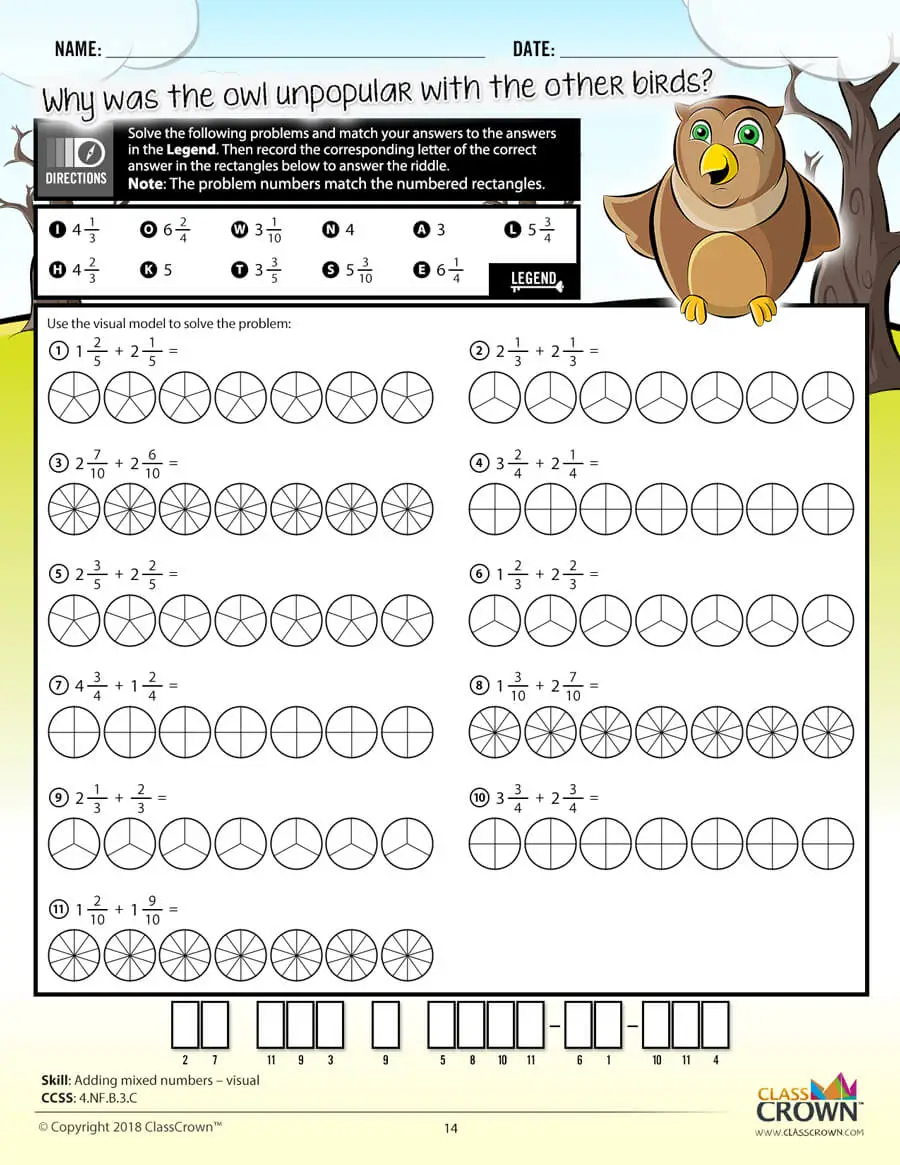## 4th Grade Math Worksheets: Pack 3

Skills included in this pack:
• Estimating capacity, American
• Estimating length, American
• Conversion tables
• Estimating length, metric
• Measuring in centimeters
• Estimating weight, American
• Comparing weight, metric
• Determining change, multiple costs
• Determining change, one cost, multiple items
• Solving word problems involving distance
• Solving word problems involving intervals of time
• Solving problems involving liquid volumes
• Solving word problems involving mass
• Finding area of rectangles
• Finding perimeter of rectangles
• Finding area of rectangles, word problems
• Finding perimeter, word problems
• Interpreting line plots that include fractions
• Interpreting line plots that include fractions
• Estimating angle measurement
• Determining if an angle is acute, obtuse, right, or straight
• Determining angles using a protractor
• Determining value of unknown angles
• Comparing angles to 90 degrees
• Determining if an angle is acute, obtuse, right, or straight
• Finding angles within shapes
• Determining parallel, perpendicular, or intersecting lines
• Determining if a triangle is right
• Determining symmetry
+ See More
Free Sample Pages Available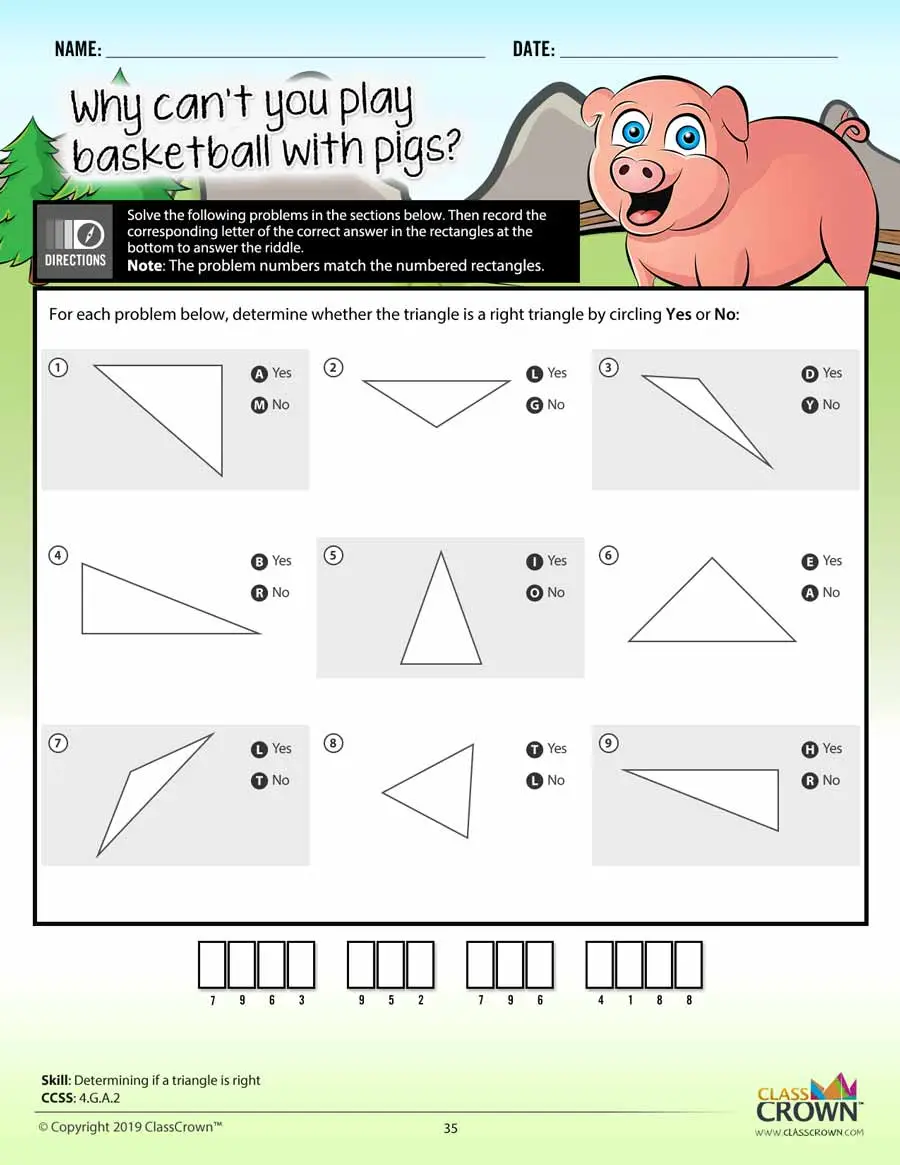## Decimals Worksheets: Pack 1

Skills included in this pack:
• Naming place value to the thousandths place
• Decimals expressed as pictures
• Decimals expressed in words
• Rounding decimals to the hundredths place
• Identifying decimals on a number line
• Comparing decimals
• Decimals expressed as fractions
• Comparing decimals with fractions
• Subtracting decimals
• Adding and subtracting decimals word problems
• Multiplying decimals by powers of 10
• Multiplying decimals by one-digit whole numbers
• Multiplying decimals by two-digit whole numbers
• Representing multiplication of decimals visually
• Multiplying decimals
• Dividing decimals by powers of 10
• Dividing decimals by whole numbers
• Dividing with decimals in the divisor
• Division: adding a decimal and zeros to the dividend
+ See More
Free Sample Pages Available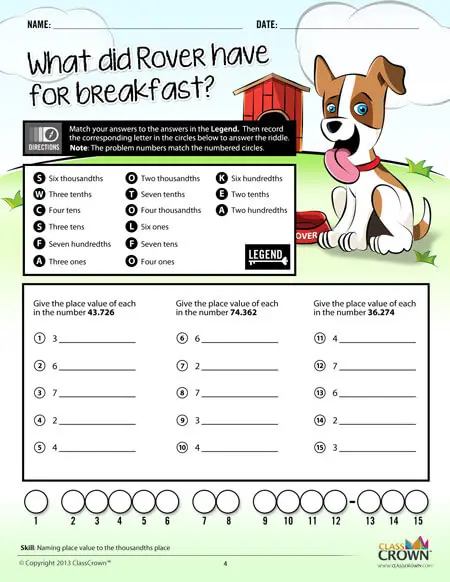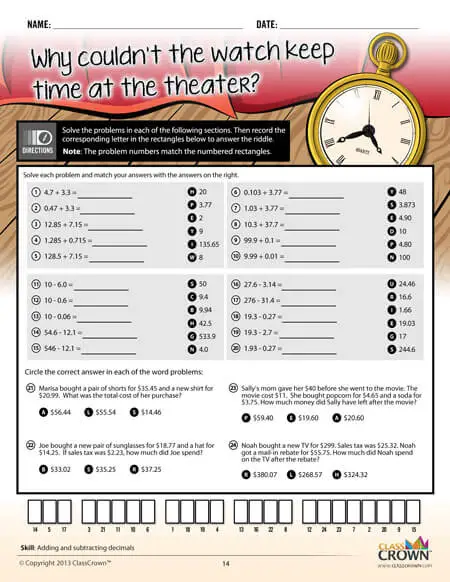## Fractions Pack 1: Concepts

Skills included in this pack:
• Identifying prime and composite numbers
• Prime factorization
• Prime factorization with exponents
• Using divisibility rules
• Using divisibility rules in word problems
• Finding greatest common factor
• Identifying equivalent fractions
• Simplifying fractions
• Converting improper fractions into mixed numbers
• Converting mixed numbers into improper fractions
• Identifying fractions on a number line
• Comparing fractions
• Comparing decimals and fractions
• Converting between decimals and fractions
+ See More
Free Sample Pages Available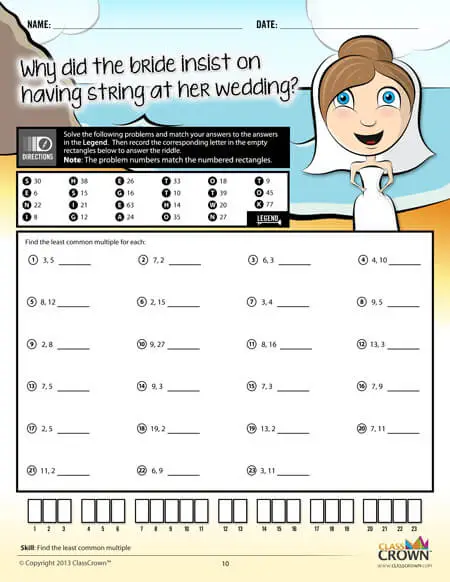## Fractions Pack 2: Adding & Subtracting

Skills included in this pack:
• Adding fractions with like denominators
• Adding mixed numbers with like denominators, no regrouping
• Adding fractions with unlike denominators
• Subtracting fractions with like denominators
• Subtracting mixed numbers with like denominators
• Subtracting fractions with unlike denominators
• Adding mixed numbers with unlike denominators
• Subtracting mixed numbers from whole numbers
• Subtracting fractions with unlike denominators and with regrouping
• Adding and subtracting fractions word problems
• Adding and subtracting mixed numbers word problems
• Adding/subtracting fractions and mixed numbers
• Adding three or more fractions with unlike denominators
• Inequalities with adding and subtracting fractions
• Estimating sums of fractions
+ See More
Free Sample Pages Available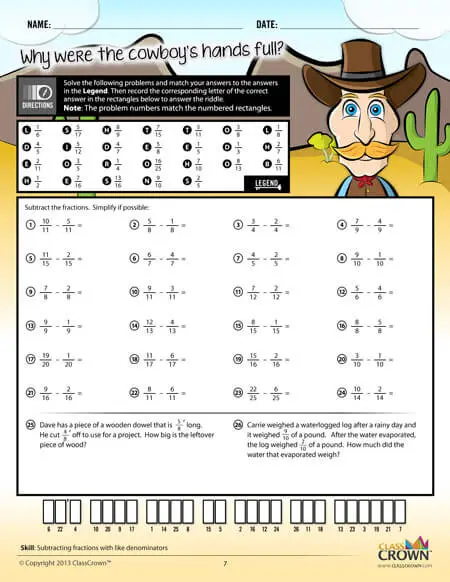## Fractions Pack 3: Multiplying & Dividing

Skills included in this pack:
• Multiplying whole numbers by fractions
• Multiplying whole number by fractions, word problems
• Representing multiplication of fractions visually
• Multiplying two fractions
• Multiplying two fractions, word problems
• Multiplying three fractions
• Multiplying a mixed number by a whole number
• Multiplying mixed numbers and fractions
• Multiplying two mixed numbers
• Dividing whole numbers by fractions
• Dividing fractions by whole numbers
• Dividing two fractions
• Dividing fractions and mixed numbers
• Dividing two mixed numbers
• Multiplying and dividing fractions and mixed numbers, word problems
+ See More
Free Sample Pages Available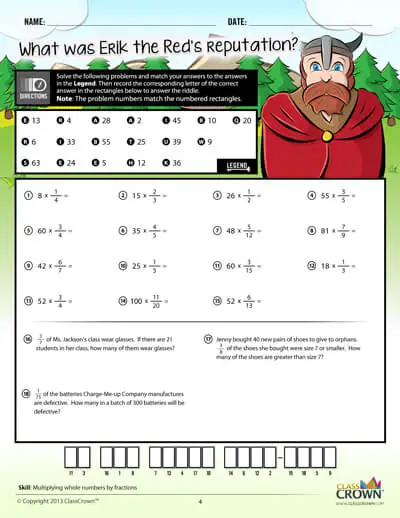## Geometry Worksheets: Pack 1

Skills included in this pack:
• Identifying planar figures
• Identifying a shape/figure when described
• Identifying types of triangles as scalene, isosceles, or equilateral
• Identifying similar and congruent figures
• Identifying nets
• Identifying acute, obtuse, and right angles
• Identifying acute, obtuse, and right triangles
• Using a protractor to find the measure of an angle
• Estimating angle measures
• Finding missing angles using properties of adjacent angles
• Identifying lines of symmetry
• Identifying lines, line segments, and rays
• Identifying parallel, perpendicular, and intersecting lines
• Identifying rotations, reflections, and translations
• Finding unknown angles in triangles and quadrilaterals
• Identifying complementary and supplementary angles
• Identifying number of sides, vertices, edges, and faces of 3-dimensional figures
+ See More
Free Sample Pages Available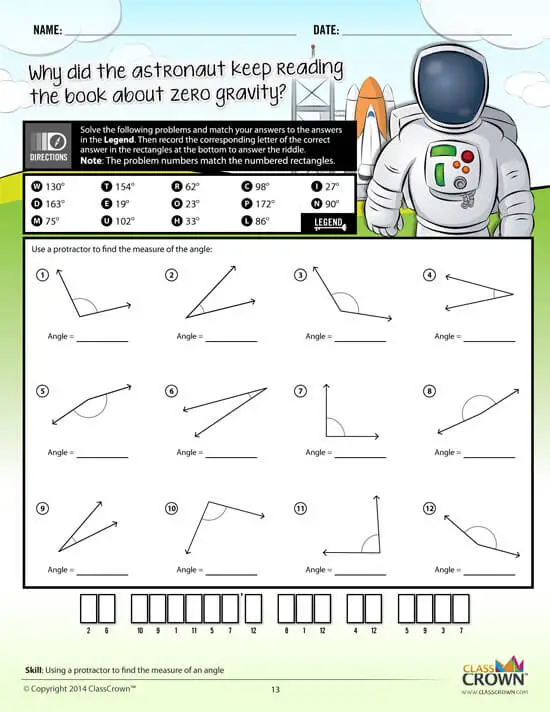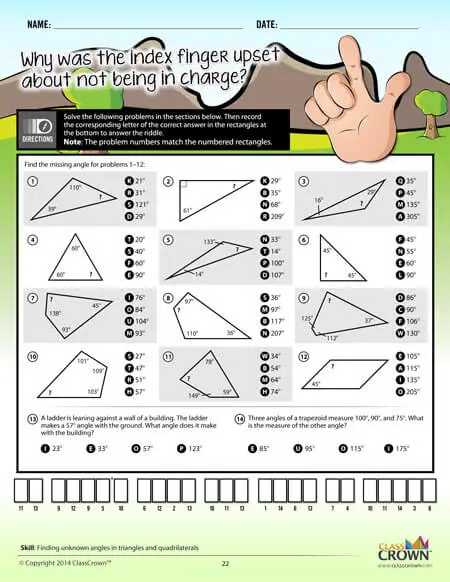Self Checking

Our math worksheets introduce a puzzle aspect to math, giving students immediate feedback as to whether or not they are solving problems correctly. If the answer to the riddle isn't spelled correctly, the student knows which problems he's made an error on.Fun Puzzle AspectImmediate Feedback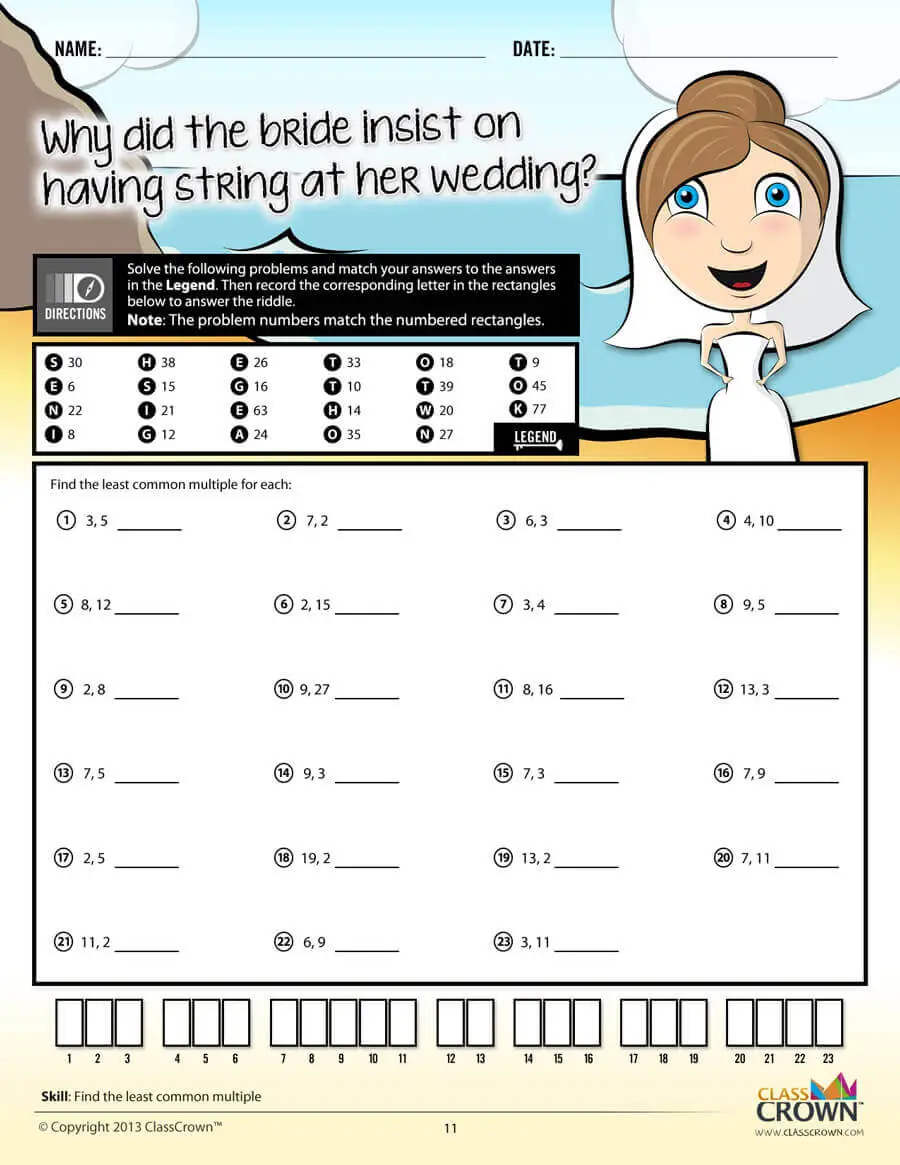Problem Solving Motivation

Each math worksheet contains a riddle that the student solves by completeing all the problems on the worksheet. This keeps kids motivated to complete each problem so that they can find the answer to the riddle.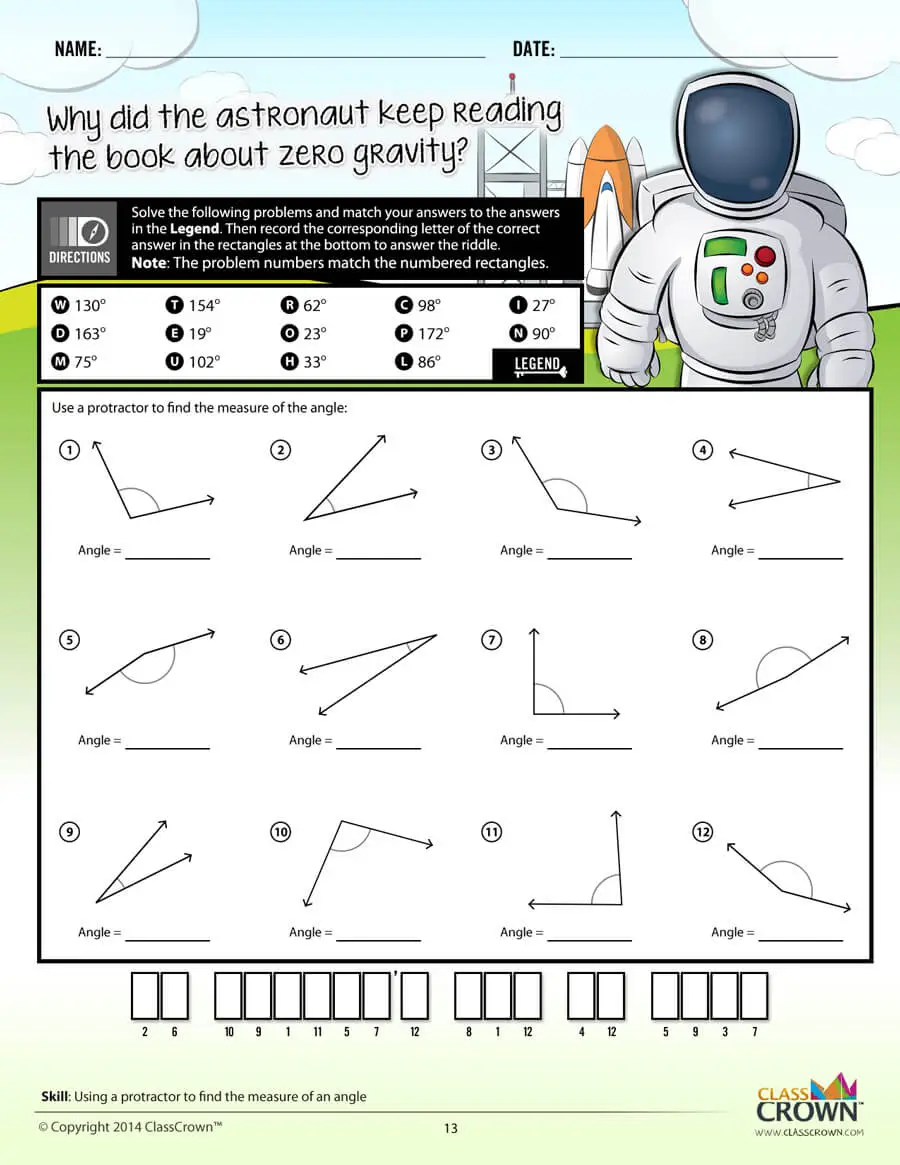Common Core Aligned

All our math worksheet packs are designed with Common Core in mind. That way you don’t have to worry about whether your math ciriculum is aligned or not when you incorpoate ClassCrown Riddle-Me-Worksheets in your lesson plans.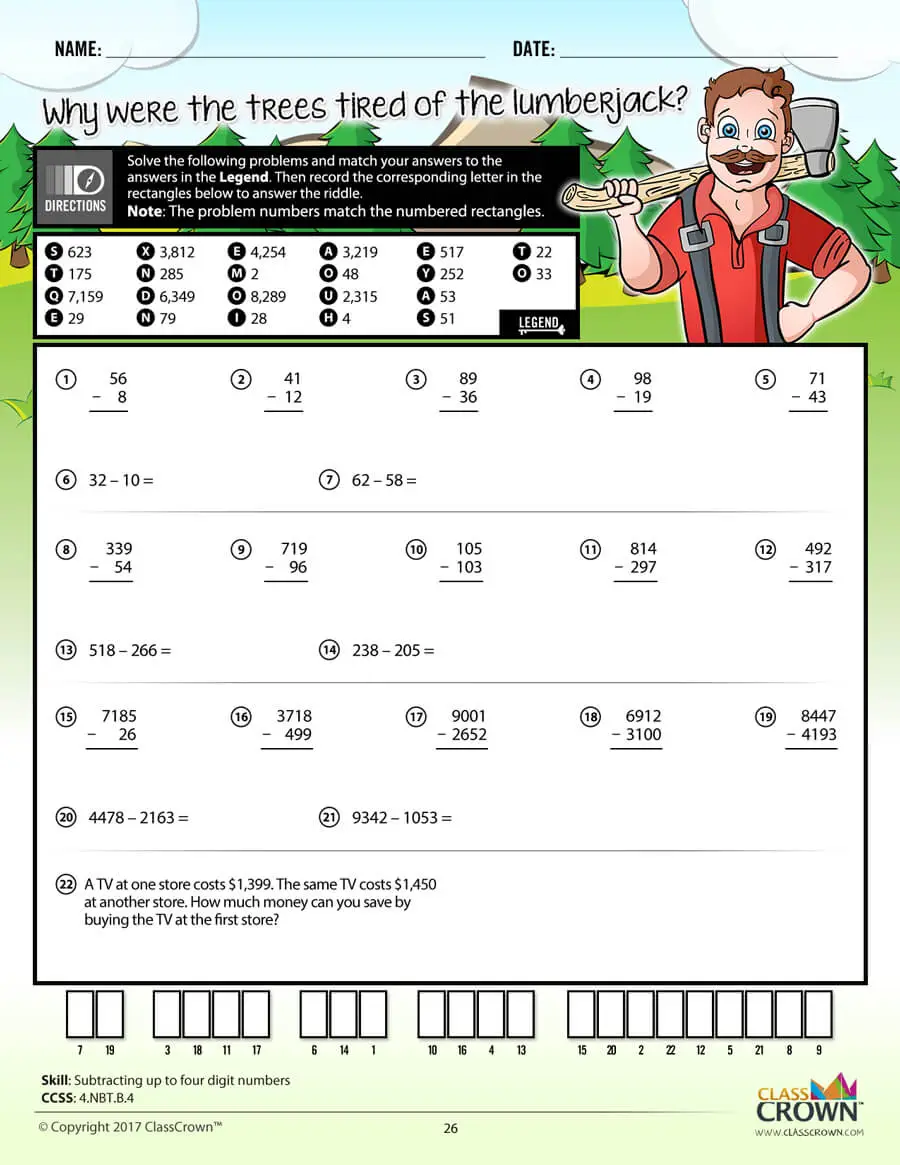High Quality Design

Each page of our math worksheets has been produced in high resolution at 144 dpi and designed in full, vibrant color for maximum quality. They look stunning whether you are printing in color or black and white.High Resolution
(144 dpi)Stunning Color & Clarity